# Domain of holomorphy

A domainin a complex spacefor which there exists a function, holomorphic in, that is not holomorphically extendable to a larger domain; the domainis then called the natural domain of definition of. For example, the natural domain of definition of the functionis the unit disc, which is thus a domain of holomorphy in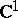. Any domain inis a domain of holomorphy. In,, on the contrary, not all domains are domains of holomorphy. E.g., no domain of the form, whereis a compactum contained in, is a domain of holomorphy.

A domain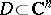is said to be holomorphically convex if for each compact set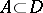there exists a compact set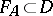containingsuch that for any pointthere exists a holomorphic functioninsuch thatA domainis a domain of holomorphy if and only if it is holomorphically convex (the Cartan–Thullen theorem). A domainis a domain of holomorphy if and only if each point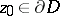has a barrier — a holomorphic function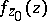inthat cannot be holomorphically continued to. For example, ifis an arbitrary domain in, then the functionis a barrier at any point, so thatis a domain of holomorphy; ifis a convex domain inand if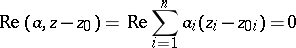is the supporting plane at a point, then the function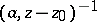is a barrier at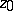, and for this reason any convex domain inis a domain of holomorphy.

The intersection of domains of holomorphy is a domain of holomorphy; any biholomorphic mapping maps a domain of holomorphy onto a domain of holomorphy (the Behnke–Stein theorem).

A domainis said to be pseudo-convex if the functionis a plurisubharmonic function in, where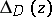is the distance from the pointto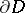. A domain is a domain of holomorphy if and only if it is pseudo-convex (Oka's theorem). That, in Oka's theorem, this condition is sufficient, forms the content of the Levi problem, formulated by E. Levi in 1911. It was solved by K. Oka in 1942 for; it was solved independently by Oka, F. Norguet and H. Bremermann in 1953–1954 for.

A domain of holomorphy with a sufficiently smooth boundary can be locally described. A domainis said to be pseudo-convex at a pointif there exists a neighbourhoodofand a real-valued functionof class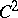such that: a)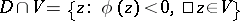; and b) on the plane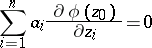the Hessian form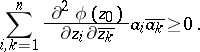If in condition b) strict inequality holds for all vectorsunder consideration, the domainis said to be strictly pseudo-convex at the point. A domainis said to be (strictly) pseudo-convex in the sense of Levi if it is (strictly) pseudo-convex at all points.

If a domain is strictly pseudo-convex in the sense of Levi, it is pseudo-convex (Levi's theorem).

The domain of holomorphy of a function, defined in an initial neighbourhood, can be constructed by expansions into Taylor series using the principle of holomorphic continuation; it may then turn out that in the domain thus constructed the holomorphic functionis not single-valued. In order to make the function single-valued, the concept of a domain must be widened. This is done by the introduction of a Riemann (Riemannian) domain (a covering domain, a multi-sheeted domain) over(a Riemann domain overis known as a Riemann surface). The concept of a domain of holomorphy is generalized to Riemann domains and even to objects of a more general structure — complex manifolds and complex spaces. The generalization of the concept of a domain of holomorphy leads to Stein spaces (cf. Stein space).